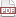### Tip 28: Vectorize a function

26 November 11. [link]PDF version

level: easy if you've read all the medium tips so far
purpose: make your code and math look more similar

Part of a series of tips on POSIX and C. Start from the tip intro page, or get 21st Century C, the book based on this series.

The free function takes exactly one argument, so we often have a part at the end of a function of the form

free(ptr1);
free(ptr2);
free(ptr3);
free(ptr4);

!How annoying! No self-respecing LISPer would ever allow such redundancy to stand, but would write a macro to allow a vectorized free function that would allow:
free_all(ptr1, ptr2, ptr3, ptr4);


If you've been following along since Tip 24, then the following sentence will make complete sense to you: we can write a variadic macro that generates an array (ended by a stopper) via compound literal, then runs a for loop over the array. Here's the code:

#define fn_apply(fn, ...) { \
void *stopper_for_apply = (int[]){0};    \
void **list_for_apply = (void*[]){__VA_ARGS__, stopper_for_apply}; \
for (int i=0; list_for_apply[i] != stopper_for_apply; i++) fn(list_for_apply[i]);\
}


We need a stopper that we can guarantee won't match any in-use pointers, including any NULL pointers, so we use the compound literal form to allocate an array holding a single integer, and point to that. Notice how the stopping condition of the for loop looks at the pointers themselves, not what they are pointing to.

Usage so far:

fn_apply(free, ptr1, ptr2, ptr3, ptr4);


If we want this to really look like a function, then we can do that via one more macro:

#define free_all(...) fn_apply(free, __VA_ARGS__);

//We can wrap this foreach around anything that takes in a pointer.
//For GSL and Apophenia users, let's define:
#define gsl_vector_free_all(...) fn_apply(gsl_vector_free, __VA_ARGS__);
#define gsl_matrix_free_all...) fn_apply(gsl_matrix_free, __VA_ARGS__);
#define apop_data_free_all...) fn_apply(apop_data_free, __VA_ARGS__);


#include <stdio.h>
#define fn_apply(fn, ...) { \
void *stopper_for_apply = (int[]){0};    \
void **list_for_apply = (void*[]){__VA_ARGS__, stopper_for_apply}; \
for (int i=0; list_for_apply[i] != stopper_for_apply; i++) fn(list_for_apply[i]);\
}

#define free_all(...) fn_apply(free, __VA_ARGS__);

int main(){
double *x= malloc(10);
double *y= malloc(100);
double *z= malloc(1000);

free_all(x, y, z);
}
`

If the input isn't a pointer but is some other type (int, float, &c), then you'll need a new macro. Implementing this (either for one new type or taking a type as an argument to the macro) is left as an exercise for the reader. I showed you a version with strings in the last tip. Also, if you want compile-time warnings about type errors, then you can rewrite the function here to use gsl_vector * pointers, gsl_matrix * pointers, ..., instead of just void* pointers.

You could rewrite this to return a value for each input item, but at this point we might be stretching what we want a macro to do. If you're a fan of the Apophenia library, it has the apop_map function (and friends) to do this sort of thing with vectors and matrices.